# Economic Data Analysis2

[From
Bjorn Simonsen (2004.02.18.15:00 EuST)]

[From Rick Marken (2004.02.04.1025)]

Ø
The result
shows what appears to be a clear positive relationship between
discount

Ø
rate and inflation rate, exactly the opposite of the relationship
assumed in popular

Ø
discussions of economic policy.

To the right you see the graph from
Norwegian data.

[insert
inflatedisc.jpg here]

The positive
relationship between discount rate and inflation rate shows up at nearly

all phase delays between
these time plots of these two variables. This is shown in the

lagged analysis in the
attached laginflatedisc.jpg. The lags/leads on the X axis are the

number of months by
which the discount rate leads (- values) or lags (+ values) inflation

rate. Discount rate
does not become negatively related to inflation until 22 months before

the inflation changes.
And the negative relationship is quite low. The negative relationship

between discount rate
and inflation never exceeds -.11, and that that level of negative

relationship doesn’t
occur until discount rate leads inflation by 36 months (3 years).

Compare this to the
very strong positive relationship (.59) between discount and inflation

rate that reaches a
maximum when discount rate follows inflation rate within 11 months.

2004.02.11. I have till now spent some time on this thread and I will give you
some comments. I have collected the same data as you did and I have analysed

I don’t agree completely with your
formula “ ((CPI(t)-CPI(t-1))/CPI(t).) is the way the government
measures inflation rate”. I think divisor shall be CPI(t-1), because the change
is from time (t-1). And the formula
((CPI(t)-CPI(t-1))/CPI(t-1)). I thought that this should give growth
when you have decrease and reversed, but I can’t see any differences in my
graphs in contrast to yours. Maybe you have used ((CPI(t)-CPI(t-1))/CPI(t-1))
when you calculated yourself?

Here is
my graph where I show the Correlation coefficients phase leaded/lagged. It is Quite as yours.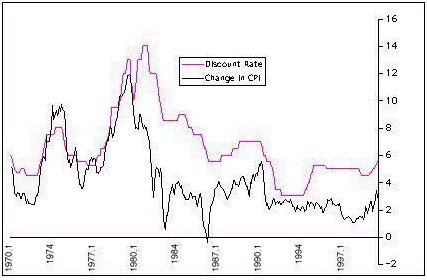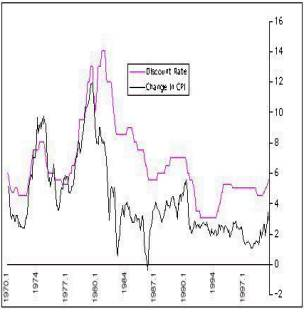image003.wmz (4.27 KB)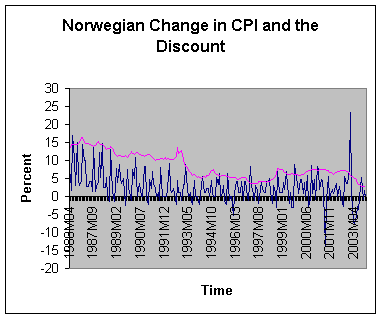image005.wmz (2.07 KB)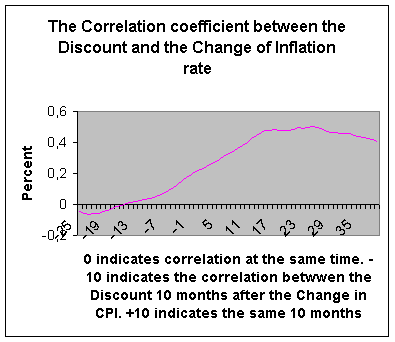image007.wmz (1.56 KB)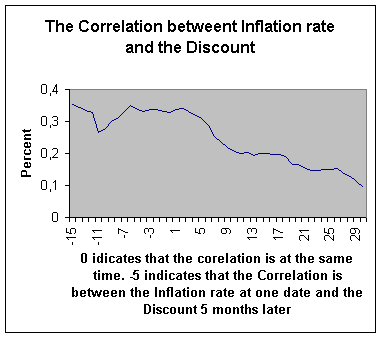image009.wmz (2.23 KB)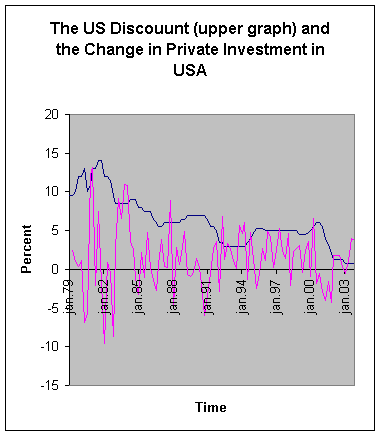image011.wmz (1.28 KB)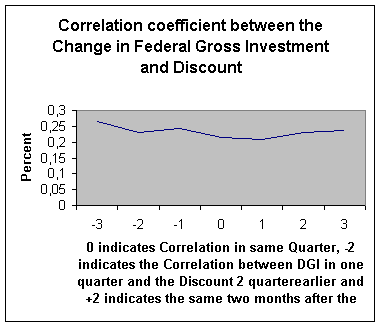image013.wmz (6.28 KB)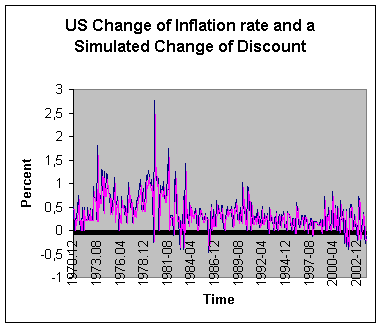image015.wmz (4.36 KB)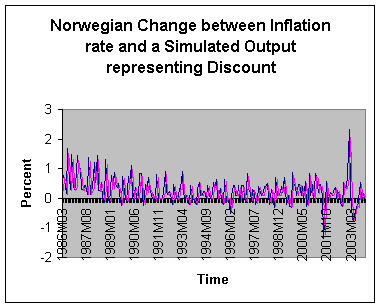image017.wmz (4.37 KB)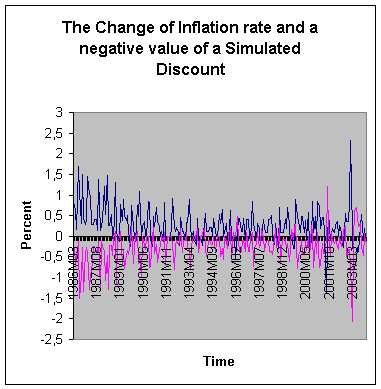oledata2.mso (305 KB)

···

To
the left you find my graph from US data. The graph is quite like yours of
course. To the right you see a different graph. Here is no negative data at
all.

Ø
………This results in the positive correlation between
discount and feedback when

Ø
discount follows inflation (positive lag values). But changes
in the discount rate seem

Ø
to be positively related to inflation (rather than
negatively, as assumed by the Fed) as

Ø
indicated by the positive relationship between
discount and inflation when discount

Ø
precedes inflation (negative lag values). This
positive feedback relationship between

Ø
the Fed chief’s actions and the results of those
actions on the controlled variable

Ø
inflation – would result in runaway inflation
if the Fed chie kept raising rates. But

Ø
the positive relationship between the Fed chief
actions and inflation seems to level

Ø
off after a few months. So runaway inflation is
prevented by the limit to the Fed chief

Ø
response to the inflation he has coaused causes by his
own actions.

I
think there is a positive
correlation between Discount
and the Inflation rate when the Discount is correlated with the Inflation rate some months before. But
the value is low, and it doesn’t tell us much.

Also I see the positive feedback between
the Discount and the effect on inflation and I think the Fed chief has
something to learn about negative feedback.

Ø
Increases in the Fed discount rate takes money out of
the economy, which should make

Ø
it difficult to get money for investment and growth.
My own visual inspection of the data

Ø
led me to the same conclusion: it looks like discount
rate and growth are negatively

Ø
related. But I wanted a quantitative measure so I got
the raw data and made my

Ø
own version of Canterbury’s Figure 14.2, which is the
graph is seen in growdisc.jpg.

Ø
I used the raw data (actually, I was able to get data
that went back to 1959, rather

Ø
than just 1975, but the statistical results are the
same for the 1959-2000 and for the

Ø
1975-2000 time period) to compute the actual
correlation between discount rate and

Ø
growth. And the results were a big surprise. It turns
out that the zero lagged correlation

Ø
between discount and growth is positive (.25). The
apparent negative relationship

Ø
between the discount and growth curves seen in
growdisc.jpg is actually an optical

Ø
illusion. The complete phase analysis of the
relationship between discount and growth

Ø
is shown in the graph in laggrowthdisc.jpg.

I did the same analyse as you and my graph
is quite like your laggrowthdisc.jpg. Below my graph.

But
my graph presents the Correlation coefficient for different Phase lags/leads
some different from your laggrowthdisc.jpg. My graph is below.

I
have tried to reanalyse my calculations and I shall do it again. I have used
the formula 100*(C21-C20)/C20 in Excel where the date in row 20 is lower than
the date in row 22.

But
both graphs indicate that

Ø
What this analysis shows is that changes in discount
rate that precede growth are

Ø
actually positively related to growth, a result that
is, again, completely the opposite

Ø
of what many economic policy experts seem to believe
is true. Increases in discount

Ø
rate are believed to lead to decreased growth. This
would show up as a negative

Ø
relationship between discount rate and growth when
discount rate precedes growth.

Ø
In fact, just the opposite is seen.

Yes

Ø
All the results presented here describe relationships
between variables that exist as

Ø
part of a closed loop system. I think the only way to
figure out what is actually going

Ø
on – that is, to figure out how variables like
discount rate, grwoth and inflation actually

Ø
influence each other – is to build closed loop models
and try to match the behavior of

Ø
the models (over time) to the observed behavior of the
variables observed.

I
have done it and I am a little excited.

I
used your model in [From Rick Marken (2004.02.07.2330)]

p = Discount in preceding month-Actual inflation rate in the preceding

month

Discount = Discount + 0.1 * ((0.01 * (0-p)) - Discount) *dt, where dt =1
month

Here you can see the graph showing the Inflation rate (upper graph) and
the Simulated Discount (lower graph)

I calculated the
Correlation coefficient between the data for same date, and it was 0.49. Then I
calculated a Correlation coefficient between the Inflation rate and the
Simulated Discount, the inflation rate for one month earlier than the Simulated
Discount setting. Here the
Correlation coefficient is 1,00. I guess you don’t believe it. Therefore I give
you a part of my calculations

I used the
formula output = output + 0.01 * (8 * (-p) -
output)

You used the
formula p = sim_discount (i-lag) -
dCPI (i-lag)

I used the
formula p = sim_discount - dCPI (i-lag)

CPI
Date
dCPI Simulated Discount

39,8

1970.12

0,50505051

0,45094052

39,8

1971.01

0

0,44866304

39,9

1971.02

0,25125628

1,5231E-07

40

1971.03

0,25062657

0,2232043

40,1

1971.04

0,25

0,2226449

40,3

1971.05

0,49875312

0,22208828

40,6

1971.06

0,74441687

0,44306874

40,7

1971.07

0,24630542

0,66130476

40,8

1971.08

0,24570025

0,21880619

40,8

1971.09

0

0,21826859

40,9

1971.10

0,24509804

1,5231E-07

40,9

1971.11

0

0,21773361

41,1

1971.12

0,48899756

1,5231E-07

The dCPI is calculated
as (A35-A34)/A34 ex. Line 3 an 4 (40-39.9)/39.9 = 0.25062657

And at line 4 I have
calculated the Simulated Discount as D35+0.001*(0.25*(0-(D34-C34))-D35). Here
the Correlation coefficient taken at the same date is 0.08. But if you Phase
displace one month the dCPI and the Simulated Discount 1 month later you get a
Correlation coefficient at 1.00. Try it.

I have worked with a
simulated Discount using your formula “output = output + 0.01 * (8 * (-p) -
output)” on Norwegian data. The graph below shows the Norwegian Inflation rate
and a Simulated Discount.

I calculated the
Correlation coefficient between the data for same date, and it was 0.20. Then I
calculated a Correlation coefficient between the Inflation rate and the
Simulated Discount, the inflation rate for one month earlier than the Simulated
Discount setting. Here the
Correlation coefficient is 1,00. I guess you don’t believe it. Therefore I give
you a part of my calculations

Here I used the same
formula you used output = output + 0.01 * (8 * (-p) -
output)

CPI
Date
dCPI Discount Change of Simulated Change of the Discount

The Discount

64,5

1986M03

0,78125

13,37

-1,32841328

0,278648555

64,9

1986M04

0,62015504

13,65

2,09424084

0,694444444

65

1986M05

0,1540832

14,36

5,2014652

0,551248923

66,1

1986M06

1,69230769

14,31

-0,34818942

0,136962849

66,8

1986M07

1,05900151

14,58

1,88679245

1,504273504

67

1986M08

0,2994012

14,56

-0,13717421

0,941334678

68

1986M09

1,49253731

14,46

-0,68681319

0,266134398

68,3

1986M10

0,44117647

14,6

0,96818811

1,326699834

68,5

1986M11

0,29282577

15,02

2,87671233

0,392156863

68,8

1986M12

0,4379562

16,4

9,18774967

0,260289572

69,8

1987M01

1,45348837

16,34

-0,36585366

0,389294404

70,5

1987M02

1,00286533

15,09

-7,6499388

1,291989664

The dCPI is calculated
as (A35-A34)/A34 ex. Line 4 an 5 (40-39.9)/39.9 = 0.25062657

And at line 5 I have
calculated the Simulated Discount as F20+0.01*(8*(0-(F20-C19))-F20). Here the
Correlation coefficient taken at the same date is 0.00. But if you Phase
displace one month, the dCPI and the Simulated Discount 1 month later, you get
a Correlation coefficient at 1.00. Try it.

I will show you a wonderful
figure from my Norwegian data.

This is how the
Norwegian Bank (Norwegian FED) would like to see the graph of the Inflation
rate and the Change of Discount.

The Correlation
coefficient with 1-month phase shift is –1.00, telling me that their action with the discount is exact
opposite the Inflation rate. When the inflation rate grows they choose a
Discount with correct effect.

I don’t believe this
is true. I have tried to extricate myself and find where I do something wrong. But

Have a nice weekend.

bjorn

[From Rick Marken (2004.02.21.0930)]

Bjorn Simonsen (2004.02.18.15:00 EuST)--

Rick Marken (2004.02.04.1025)--

The result shows what appears to be a clear _positive_ relationship between discount
rate and inflation rate, exactly the opposite of the relationship assumed in popular
discussions of economic policy.

To the right you see the graph from Norwegian data.

This is great stuff, Bjorn. Thanks.

I dont agree completely with your formula ((CPI(t)-CPI(t-1))/CPI(t).) is the way the government measures inflation rate. I think divisor shall be CPI(t-1), because the change is from time (t-1).

I think you're right. I'll change it, but I don't think it will make much of a difference. Even looking at inflation as a pure differential (CPI(t)-CPI(t-1)) didn't change the model predictions much. But I think it is good for us to be consistent with the standard calculations of things like growth and inflation.

Here is my graph where I show the Correlation coefficients phase leaded/lagged. It is Quite as yours.

Yes. Very nice! It is really great that you are doing this. Thank you!!

Could you do me a favor and send me (in a spreadsheet) the Norwegian data on gross private investment and growth in GDP (or GNP) going back as far as you can go. (I got back to 19653). I used quarterly data but I'll take what I can get. What I would like is real (not inflation adjusted) GDP (or GNP), inflation adjusted GDP (or GNP) and real (not inflation adjusted) gross private investment (I don't think they report inflation adjusted investment, I presume because they assume it will be measured as a proportion of GNP, which would also have to be in real dollars). If you can get me gross government investment as well that would be great (in the US they report defense and domestic investment as separate parts of governement investment).

I would love to see whether the results with the Norwegian data match my results with the US data. That is, I wonder if the lagged correlation between gross private investment and growth rate is actually negative (as it is for the US data) when investment leads growth by a quarter or more. My prediction is that the relationship between investment and growth will be the same for all countries regardless of the nature of their economic system, although I suppose that gross private investment would not exist as a line item but but would be part of gross government investment in pure communist countries, like Cuba and Vietnam. I predict that even in communist countries the negative relationship between prior investment and subsequent growth will exist.

And at line 5 I have calculated the Simulated Discount as F20+0.01*(8*(0-(F20-C19))-F20). Here the Correlation coefficient taken at the same date is 0.00. But if you Phase displace one month, the dCPI and the Simulated Discount 1 month later, you get a Correlation coefficient at 1.00. Try it.

I will try it (probably won't have time until tomorrow) but I will definitely try to figure out why you get a correlation of 1.0. I think the model output must just be a delayed version of the actual data.

I will show you a wonderful figure from my Norwegian data.

That is gorgeous. Yes. It's how the US bank would like to think it works, also.

Have a nice weekend.

You have already made it very nice, indeed. If you are able to send me the Norwegian data on investment and growth that would be superb. It would be a wonderful birthday present (my birthday is next Saturday, 2/28).

Thanks again, Bjorn.

Best regards

Rick

···

--
Richard S. Marken
Home 310 474-0313
Cell 310 729-1400

[From Rick Marken (2004.02.22.1040)]

Bjorn Simonsen (2004.02.18.15:00 EuST)--

I used your model in [From Rick Marken (2004.02.07.2330)]

I calculated the Correlation coefficient between the data for same date, and it was 0.49. Then I calculated a Correlation coefficient between the Inflation rate and the Simulated Discount, the inflation rate for one month earlier than the Simulated Discount setting. Here the Correlation coefficient is 1,00. I guess you dont believe it. Therefore I give you a part of my calculations

I used the formula output = output + 0.01 * (8 * (-p) - output)

You used the formula p = sim_discount (i-lag) - dCPI (i-lag)

I used the formula p = sim_discount - dCPI (i-lag)

Did you do these calculations using Visual Basic? In my calculations, the value of sim_discount that is used in the calculation of p is the value of output calculated on the prior iteration of the following loop (lines stating with an apostrophe are remarks):

'My data started in row 5 so the loop allows for lags of -3, which would be leads of 3 months
For i = 8 + lag To 579
'The indexes on the Cells(i,j) statement and row, column
p = Cells(i - lag, 4) - Cells(i - lag, 2)
'Note that Cells(i - lag, 4) is the value of output from the prior iteration
output = output + 0.01 * (8 * (-p) - output)
Cells(i, 4) = output
Next i

The values in Cells(i,4) are the calculated discount rates (output) and the values in Cells(i,2) are actual inflation rates (dCPI). So sim_discount is the simulated discount rate setting (the Fed "move") that is made on the basis of the difference between (sim) discount rate and inflation rate (Cells(i - lag, 4) - Cells(i - lag, 2)) from the previous month (assuming lag = 1) . If you are not storing the current output as the sim_discount to be used in the calculation of p on the next trial, then you might be getting the correlation of 1.0 because you are do the lagged correlation between dCPI and a linear transformation of dCPI, represented by the equation

output = output + 0.01 * (8 * (-p) - output).

The correlation between x and ax+b is 1.0.

I look forward to getting the Norwegian data on investment and growth. I see that you did do an analysis of Federal Gross Investment and Discount Rate (something I did not do). Was this for the Norwegian data? If so, then you can apparently get the data I want: Norwegian Gross Federal and Gross Private Investment as well as Norwegian Real and Inflation Adjusted GDP.

I hope this helps.

Best regards

Rick

···

---
Richard S. Marken